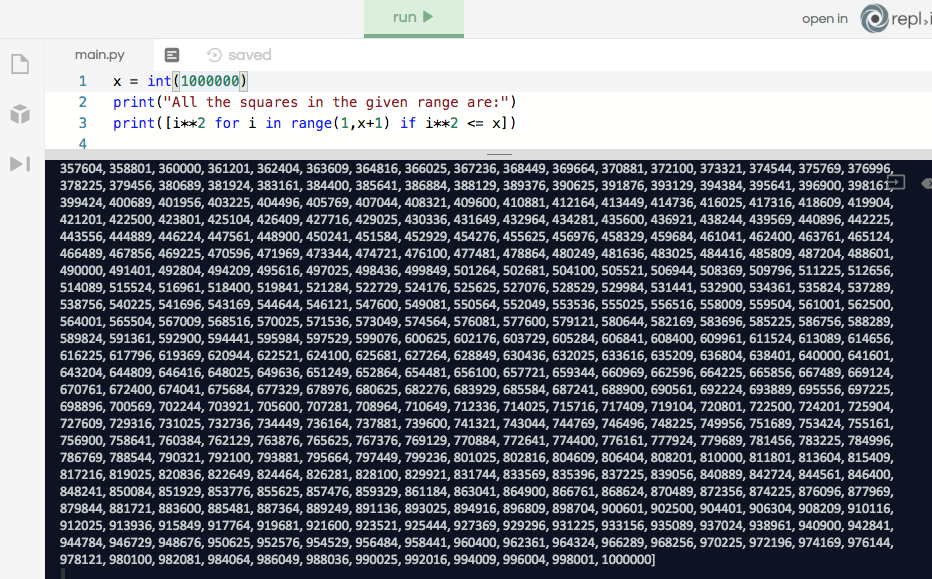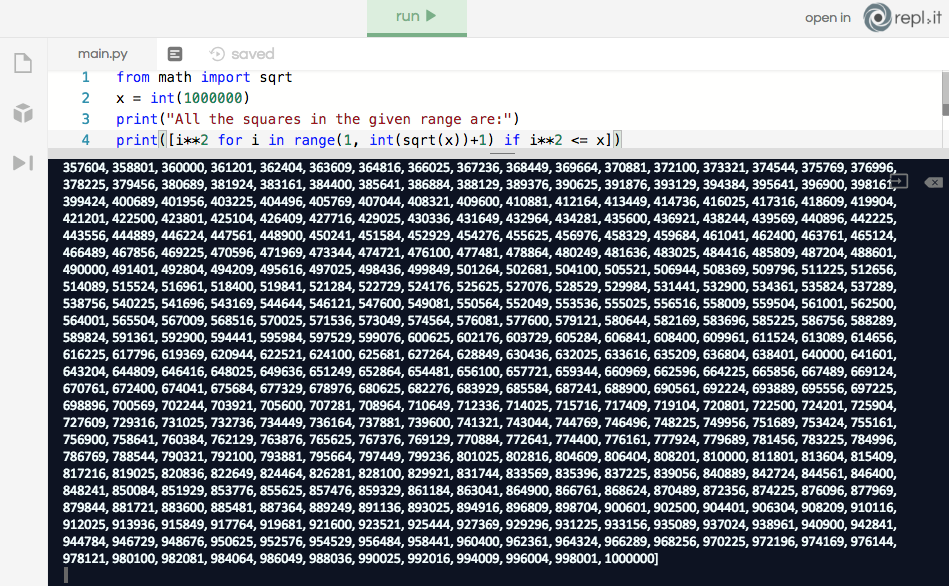6.1. While: List of squares

"For a given integer N, print all the squares of positive integers where the square is less than or equal to N, in ascending order."

Example input
50

Example output
1 4 9 16 25 36 49

You are viewing a single comment. View All
girianshiido (1)

I think it's better to use a while loop here.
For example, if x is one million, the last number of the list will be one million, but you'll compute the square of each number from one to one million (so the last square will be one trillion).

``````x = int(input("Enter the number:"))
i = 1
while i*i <= x:
print(i*i)
i += 1``````
pole55 (82)

@girianshiido This code publishes only squares which are less than a given number. We have "if i*2 <= x" here for this. it is equal to your "while ii <= x". just try it // Link to the task: https://repl.it/student/submissions/3764967

girianshiido (1)

@pole55 what I meant is that your script is computing too much stuff for its purpose. That's because range(1, x+1) is way too big, especially if x itself is big. If x=10^10, then you only really need to stop at 10^5, because the square of this number is 10^10.
Here is a modification of your own script:

``````from math import sqrt
x = int(input("Enter the number: "))
print("All the squares in the given range are:")
print([i**2 for i in range(1, int(sqrt(x))+1) if i**2 <= x])``````

Try your script with x = 10000000000 and try this new version. You'll see the difference.

pole55 (82)

its all because of "if i**2 <= x" that tells to stop at "10^5" (in your words). without this part, it will print "10^10".

girianshiido (1)

@pole55 Did you try your code with 10000000000?
Your code doesn't tell to stop at 10^5. It'll try to compute each square from 1 to x², even if i² is already greater than x. Of course, none of these numbers will be in the list, because they don't verify the condition.

pole55 (82)

@girianshiido the result does not depend on the value of the number. they are still identical. p.s.: I use here a million cause the computer hangs trying to work with 10000000000)). in both, my and yours, cases.pole55 (82)

@girianshiido oh. now i see what you are talking about! hmm. thanks

girianshiido (1)

@pole55 I know they’re identical. On my 2011 MBA, it takes forever with your code but it works instantly with the sqrt.
By the way, I just realized I forgot to remove the unnecessary condition if i^2 <=x.

``print([i*i for i in range(1, int(sqrt(x))+1)])``